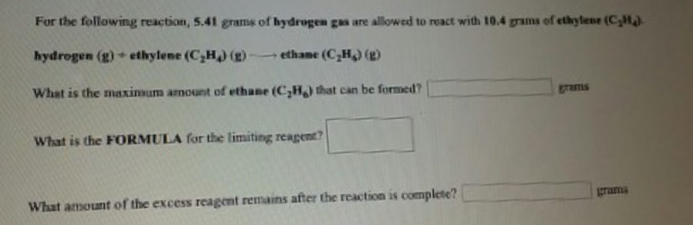# Problem: For the following reaction, 5.41 grams of hydrogen gas are allowed to react with 10.4 grams of ethylene (C2H4).hydrogen (g) + ethylene (C2H4) (g) → ethane (C2H6) (g)What is the maximum amount of ethane (C2H6) that can be formed?What is the FORMULA for the limiting reagent? What amount of the excess reagent remains after the reaction is complete?

###### FREE Expert Solution
80% (369 ratings)###### Problem Details

For the following reaction, 5.41 grams of hydrogen gas are allowed to react with 10.4 grams of ethylene (C2H4).

hydrogen (g) + ethylene (C2H4) (g) → ethane (C2H6) (g)

What is the maximum amount of ethane (C2H6) that can be formed?

What is the FORMULA for the limiting reagent?

What amount of the excess reagent remains after the reaction is complete?